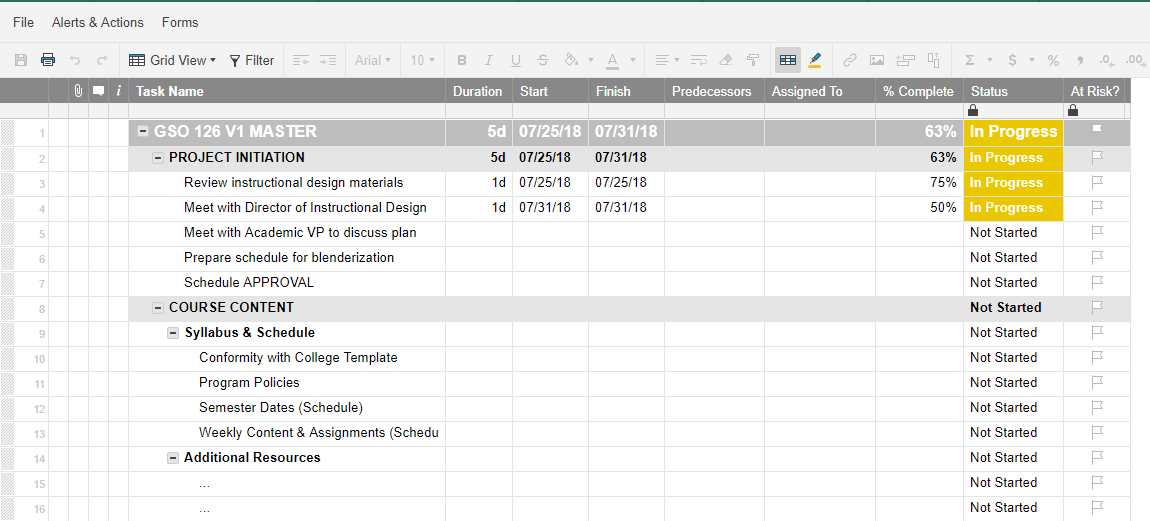or Explore Discussions

# At Risk Flag

04/11/18 Edited 12/09/19

Good Day All:

I am trying to add to the following formula below. If my Due Date (Planned) is within 1 day (i.e. due 04/12/18) and % Complete (Actual) is less than 50%, it should be Flagged.

=IF(Duration3 > 0, IF(OR(AND(TODAY() > [Due Date (Planned)]3, [% Complete (Actual)]3 < 1), AND(TODAY() > [Start Date (Planned)]3, TODAY() < [Due Date (Planned)]3, [% Complete (Actual)]3 < 0.5)), 1, 0), 0)

Thanks

• Hi Kam,

This will do what you've described:

=IF(AND(Duration3 > 0, TODAY() -1 <= [Due Date (Planned)]3, [% Complete (Actual)]3 < 0.5), 1, 0)

However, looking at your formula, it looks like you may be trying to do more than what you've indicated.

• Yes, I am trying to combine your formula with what I already have. I will try this and let you know how it works.

Thanks

• Chris:

I added your formula and it activates even if the due date is 4 days out. For example, I changed the duration to 10 days and the due date (Planned) is 04/16/18. Base on today (04/12/18), the Flag should activate on 04/13/18 if it is less than 50% complete.

Task Example: Based on the 49% and Due Date the 04/13/18. This Task should Flagged because it meets one of the conditions in the formula. Hope this helps explain it a little bit better. Thanks

MSP Issues contract amendment/PO

9d    80.0h    04/03/18    04/13/18        89%    49%

• Hi Kam,

Try: =IF(AND([Due Date (Planned)]1 <= TODAY() + 1, [% Complete]1 < 0.5), 1, 0)

Cheers,

Chris

• Chris I applied the same logic to my At Risk Column however it did not work.

At Risk Rule: If the Due Date (Planned) is 04/16/18 and %  Complete (Actual) is less than 50%. The At Risk Column should be Flagged based on the Task only has 1 day left before its due.

Formula: =IF(Duration3 > 0, IF(OR(AND([Due Date (Planned)]3 < TODAY() + 1, [% Complete (Actual)]3 < 0.5), AND(TODAY() > [Start Date (Planned)]3, TODAY() < [Due Date (Planned)]3, [% Complete (Actual)]3 = 0)), 1, 0), 0)

• Kam, IF statements read like this:

IF(a condition is true, then calculate or show this, but if it is false calculate or show this).

You can of course use as many IFs as you'd like and include boolean operators like AND/OR to create a nested IF statement. This is a little more complex and can be approached in a number of ways:

1. This method essentially asking Yes/No to get to a logical conclusion (i.e. the last False value) as every other condition is false.

=IF(condition 1 is true, then calculate or show this and stop, if condition 1 is false then evaluate IF(condition 2 is true, then calculate or show this and stop, however if condition 2 is false then evaluate IF(condition 3 is true, then calculate or show this and stop, but if condition 3 is false and therefore all conditions are false then calculate or show this)))

I find this the easiest approach for simple scenarios, as it reads like a linear story and you can easily see the logical progression. It also makes it easier to determine how many IF statements you have and therefore whether they are evaluating properly and how to close them properly (e.g. 3 IF statements = 3 closing brackets).

2. Another method is to use the true or false values to trigger subsequent IFs which allows a more dynamic (but complex) tree decision path to get to your logical conclusion.

=IF(condition 1 is true, then evaluate IF(condition 2 is true, then calculate or show this and stop, however if the condition 2 is false then evaluate IF(condition 3 is true, then evaluate IF(condition 4 is true, then evaluate IF(condition 5 is true, then calculate and or show this and stop, but if condition 5 is false then calculate or show this and stop), however if condition 4 is false then calculate or show this and stop), however if condition 3 is false then calculate or show this and stop)), however if condition 1 is false then calculate or show this and stop)

Significantly more complex to evaluate, but you can create some pretty impressive results

Looking at your requirements, I'd use method 1 to create:

=IF(AND(Duration3 > 0, OR([Due Date (Planned)]3 < TODAY() + 1, [% Complete (Actual)]3 < 0.5), TODAY() > [Start Date (Planned)]3, TODAY() < [Due Date (Planned)]3, [% Complete (Actual)]3 = 0)), 1, 0)

Your original formula had some errors amongst the OR and AND operators and you were using an additional IF where it wasn't required. You're essentially saying, If all this stuff is true (AND) including some conditions that may be one or the other (OR), then flag 1, if not flag 0.

• Very well! I appreciate all your help.

• I wonder if anyone might be able to help me with a similar problem.  I've attached a sample of a new template I'm building and I'd like the "At Risk?" flag to automatically appear if a task is between 0% (or blank) and 50% complete and the Finish date is the one day (or maybe 2) into the future, or if the task is less than 100% complete and the Finish date is in the past.  Hopefully that made sense.

I've tried several variations of the formula but none of them have worked.  I haven't included any of those iterations here because I tried so many with no idea if any of them were close.

Any ideas on how to build a formula that could accomplish the above?

Thank you!

Steve